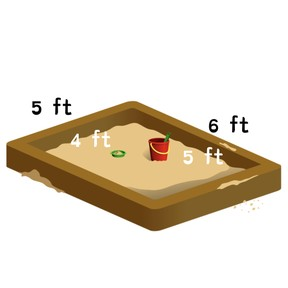Determining area

# Determining area

I can determine the area of a square or rectangle.8,000 schools use Gynzy92,000 teachers use Gynzy1,600,000 students use Gynzy

## General

Students will learn the meaning of area. They will count squares to find an area. They will also multiply length times width to determine area.

## Standards

CCSS.Math.Content.3.MD.C.7

## Learning objective

Students will be able to determine the area of a square or rectangle.

## Introduction

Students are shown various pictures of items that cover an area such as a rug or tiled wall. They discuss how these things cover a large space, or area.

## Instruction

Students learn the meaning of area as the size a surface takes up. They learn that you can find the area by counting squares. Students practice counting area this way with several shapes. Students learn that area is always measured in squares and are shown the various units that are used to measure area such as inches, feet, centimeters, or meters. Next, students are shown the length and width of the shape. They learn that you can find area faster by multiplying these two measurements. Several opportunities to practice using multiplying length times width are provided. Students also solve story problems requiring them to determine area.

## Quiz

Students respond to ten multiple-choice and true/false questions.

## Closing

Students will choose a book from their desks or around the room. They will measure to find the length and width. They will use these measurements to find the area of the book.

## Instruction materials

- ruler with inches and centimeters
- book

### The online teaching platform for interactive whiteboards and displays in schools

• Save time building lessons

• Manage the classroom more efficiently

• Increase student engagement